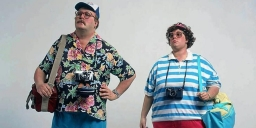# Kilometers 8343

The route of the tourist trip measures 28 cm on a map with a scale of 1:50000. The average speed of the march is 4km/h.
How many kilometers does the trip measure?
How many hours do students spend on the road?

s =  14 km
t =  3.5 h

### Step-by-step explanation:Did you find an error or inaccuracy? Feel free to write us. Thank you!

Tips for related online calculators
Need help calculating sum, simplifying, or multiplying fractions? Try our fraction calculator.
Check out our ratio calculator.
Do you want to convert length units?
Do you want to convert velocity (speed) units?
Do you want to convert time units like minutes to seconds?